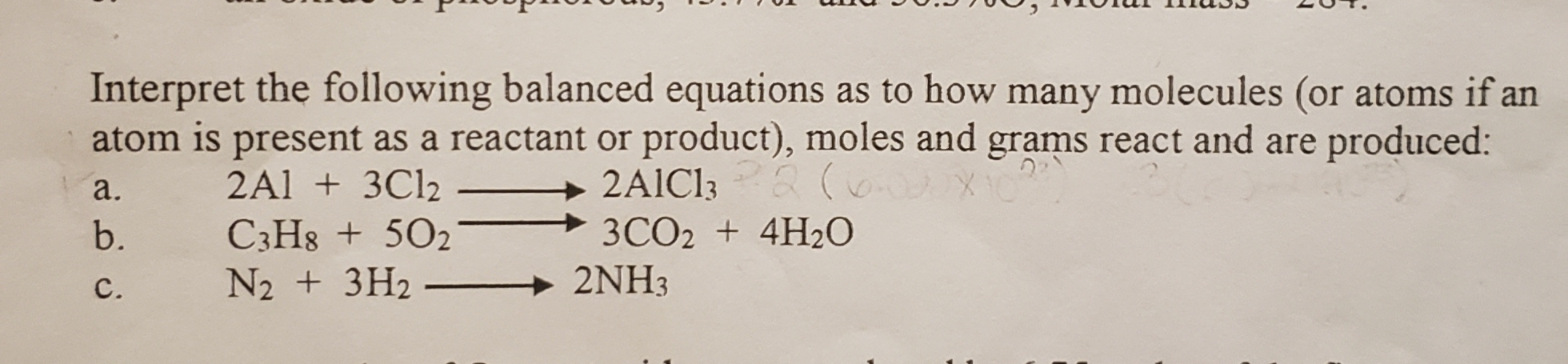Interpret the following balanced equations as to how many molecules (or atoms if anatom is present as a reactant or product), moles and grams react and are produced:a. →2AICI3b. C3H8+502 → 3CO2 + 4H2O2Al 3C1

Questionhelp_outlineImage TranscriptioncloseInterpret the following balanced equations as to how many molecules (or atoms if an atom is present as a reactant or product), moles and grams react and are produced: a. →2AICI3 b. C3H8+502 → 3CO2 + 4H2O 2Al 3C1 fullscreen
Step 1

Numbers of moles of atom or molecule are equal to atomic mass of an atom or molecular mass of molecule.

1 mole of atom/ molecule = 6.022 × 1023 atom/molecule

Number of moles = Mass / Molar mass

Consider the reaction 1:

Step 2

2 × 6.022 × 1023 atoms of Aluminum + 3 × 6.022 × 1023 atoms of Chlorine react together to produce 2 × 6.022 × 1023 molecules of AlCl3.

2 moles of Aluminum + 3 moles of Chlorine react together to produce 2 moles of AlCl3.

2 × 26.9 g of Aluminum (molar mass = 26.9 g/mol) + 3 × 35.45g of Chlorine (molar mass = 35.45 g/mol)  to produce 2 × 133.34 g of AlCl3 (molar mass = 133.34 g/mol).

Consider the reaction 2:

Step 3

1 × 6.022 × 1023 molecules of C3H8  + 5 × 6.022 × 1023 molecules of oxygen reacts together to produce 3 × 6.022 × 1023 molecules of CO2  + 4 × 6.022 × 1023 molecules of H2O.

1 moles of C3H8 + 5 moles of oxygen react together to produce 3 moles of CO2 + 4 moles molecules of H2O.

1 ...

Want to see the full answer?

See Solution

Want to see this answer and more?

Our solutions are written by experts, many with advanced degrees, and available 24/7

See Solution
Tagged in

Chemistry